Duodecimal (The Dozenal) number system Avtukh (rus)

 image of the character value of the symbol the title character compliance decimal system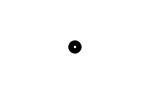zero (nothing) zero ---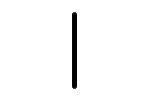one of one one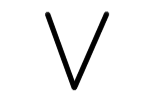two parts two two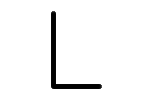three parts (a quarter) a quarter three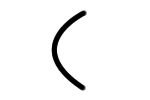four parts (one-third) one-third four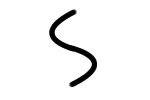five parts five five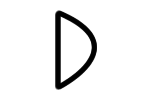six parts (half) half six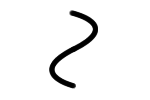seven parts seven seven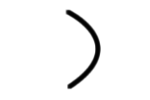eight parts (two-thirds) two-thirds eight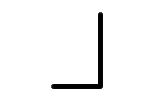nine parts (three quarters) three quarters nine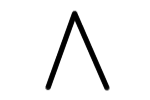ten parts ten ten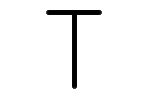eleven parts a-unit (almost unit) eleven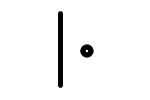twelve parts (unit) unit twelve